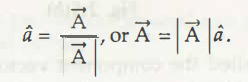It is easy to make use of vectors of unit length which can surely specify any directions of vector quantities in different coordinate systems. In Cartesian coordinates the directions are signify as x and y which is usually denoted as x^ and y^. The triangle written above the letter is referred as “hat”. In case of unit vectors related to Cartesian coordinates may describe a circle which is known as “unit circle” and this comes along with radius one.

The unit vector with any vector is calculated while making use of the formula:, or can divide vector by its magnitude.

It is necessary to take into account different possible vectors of length one along with all possible angles in coordinate system and finally place on the coordinates.

Links of Next Mechanical Engineering Topics:-### Customer Reviews

My Homework Help
Rated 5.0 out of 5 based on 510 customer reviews at